# Colour in generativepy

Martin McBride, 2020-08-31
Tags generativepy tutorial colour rgb hsl alpha css colours
Categories generativepy generativepy tutorial

generativepy provides several ways of defining colour values, via the color module. Colours are stored as Color objects.

You can create colours using:

• RGB values
• Grey values
• CSS named colours
• HSL values

All colour spaces also support transparency (alpha) values.

You can also create a new colour from an existing colour by either:

• Setting a new R, G, B, H, S, L or A value.
• Multiplying the R, G, B, H, S, L or A by a factor.

All colours are stored internally as RGBA colours, using floating point values in the range 0.0 to 1.0 to represent each channel.

generativepy functions accept Color objects. Color objects also

## RGB colours

You can create a color from RGB values using:

Color(r, g, b)


Where r, g and b are numbers. The supplied values will be clamped to the range 0.0 to 1.0.

For each colour value r, b and b, a value of zero indicates that none of that colour is present, 0.5 means the colour is present at 50% of full intensity, 1.0 means that colour is present at full intensity.

Here is some code that creates a set of RGB colours and draws filled rectangles:

from generativepy.drawing import make_image, setup
from generativepy.color import Color
from generativepy.geometry import Rectangle

def draw_rgb(ctx, width, height, frame_no, frame_count):
setup(ctx, width, height, background=Color(1))

pos = [10, 10]
w = 100
h = 100

Rectangle(ctx).of_corner_size(pos, w, h).fill(Color(0, 0, 0))
pos += w
Rectangle(ctx).of_corner_size(pos, w, h).fill(Color(0.5, 0, 0))
pos += w
Rectangle(ctx).of_corner_size(pos, w, h).fill(Color(1, 0, 0))
pos += w
Rectangle(ctx).of_corner_size(pos, w, h).fill(Color(0.5, 1, 0))
pos += w
Rectangle(ctx).of_corner_size(pos, w, h).fill(Color(0.5, 0, 1))
pos += w
Rectangle(ctx).of_corner_size(pos, w, h).fill(Color(0, 1, .5))
pos += w
Rectangle(ctx).of_corner_size(pos, w, h).fill(Color(0.5, 0.5, 0.5))
pos += w

make_image("colour-rgb.png", draw_rgb, 720, 120)


Here is the image it creates: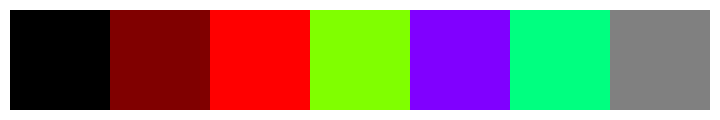The images mix various amounts of red, green and blue to create different colours. You can use this code to experiment with other combinations.

## Grey colours

Mixing equal amount of red, green and blue will give a grey colour. An rgb value of (0, 0, 0) gives black, (1, 1, 1) gives white, (0.2, 0.2, 0.2) gives a fairly dark grey, and so on.

As a convenience you can create a grey value like this:

You can create a colour from RGB values using:

Color(k)


This is effectively the same thing as Color(k, k, k).

This code creates a set of grey values from 0.0 (black) to 0.8 (very light grey):

from generativepy.drawing import make_image, setup
from generativepy.color import Color
from generativepy.geometry import Rectangle

def draw_grey(ctx, width, height, frame_no, frame_count):
setup(ctx, width, height, background=Color(1))

pos = [10, 10]
w = 100
h = 100

Rectangle(ctx).of_corner_size(pos, w, h).fill(Color(0))
pos += w
Rectangle(ctx).of_corner_size(pos, w, h).fill(Color(0.2))
pos += w
Rectangle(ctx).of_corner_size(pos, w, h).fill(Color(0.4))
pos += w
Rectangle(ctx).of_corner_size(pos, w, h).fill(Color(0.6))
pos += w
Rectangle(ctx).of_corner_size(pos, w, h).fill(Color(0.8))
pos += w

make_image("colour-grey.png", draw_grey, 720, 120)


Here is the image it creates: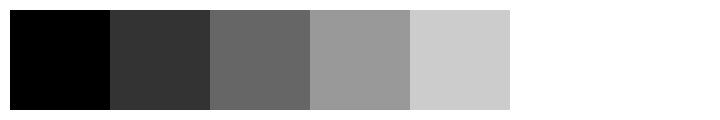## CSS named colours

The CSS named colours are a set of over 150 colours that have been carefully chosen as generally pleasing colours. They were originally intended for use on websites, but they are also good for general design as well as certain types of generative art.

The set of colours includes the standard colours such as black, white, red, magenta etc. It also includes a large number of other colours across the spectrum.

You can create a colour from CSS name using:

Color("slateblue")


You must use a valid CSS name (you can find a list on various websites, or look at the color module source code). The string is case insensitive.

In addition, you can create variants of each colour by changing the brightness or saturation, as shown later.

This code creates a set of coloured squares using a selection of name colours:

from generativepy.drawing import make_image, setup
from generativepy.color import Color
from generativepy.geometry import Rectangle

def draw_css(ctx, width, height, frame_no, frame_count):
setup(ctx, width, height, background=Color(1))

pos = [10, 10]
w = 100
h = 100

Rectangle(ctx).of_corner_size(pos, w, h).fill(Color("salmon"))
pos += w
Rectangle(ctx).of_corner_size(pos, w, h).fill(Color("gold"))
pos += w
Rectangle(ctx).of_corner_size(pos, w, h).fill(Color("seagreen"))
pos += w
pos += w
Rectangle(ctx).of_corner_size(pos, w, h).fill(Color("slateblue"))
pos += w
Rectangle(ctx).of_corner_size(pos, w, h).fill(Color("darkorchid"))
pos += w
Rectangle(ctx).of_corner_size(pos, w, h).fill(Color("hotpink"))
pos += w

make_image("colour-css.png", draw_css, 720, 120)


Here is the image it creates:## HSL colours

HSL colours define colours based on hue, saturation and lightness:

• Hue defines the basic colour. A value from 0.0 to 1.0 represents the position of the colour on a colour circle.
• Saturation determines the "purity" of the colour. A saturation of 1.0 create a pure colour. With lower saturations the colour appears more "greyed out" or pastel, but it is still identifiable as the same underlying colour. As the saturation approaches 0, the underlying colour vanishes and the result becomes grey.
• Lightness controls how light the colour is. Varying the lightness creates lighter or darker versions of the same colour. As the lightness approaches 0, the colour goes to black, and as the lightness approaches 1 the colour goes to white.

HSL is a remapping on RGB, which means that any colour you can create in RGB you can also create in HSL.

The advantage of HSL is that, once you have chosen a hue, you can easily create more or less saturated, and darker or lighter variants of the same colour, It is difficult to do that with RGB.

This code creates a three lines of colour patches that vary in hue, saturation and brightness:

from generativepy.drawing import make_image, setup
from generativepy.color import Color
from generativepy.geometry import Rectangle

def draw_hsl(ctx, width, height, frame_no, frame_count):
setup(ctx, width, height, background=Color(1))

pos = [10, 10]
w = 70
h = 100

Rectangle(ctx).of_corner_size(pos, w, h).fill(Color.of_hsl(0.0, 0.5, 0.5))
pos += w
Rectangle(ctx).of_corner_size(pos, w, h).fill(Color.of_hsl(0.1, 0.5, 0.5))
pos += w
Rectangle(ctx).of_corner_size(pos, w, h).fill(Color.of_hsl(0.2, 0.5, 0.5))
pos += w
Rectangle(ctx).of_corner_size(pos, w, h).fill(Color.of_hsl(0.3, 0.5, 0.5))
pos += w
Rectangle(ctx).of_corner_size(pos, w, h).fill(Color.of_hsl(0.4, 0.5, 0.5))
pos += w
Rectangle(ctx).of_corner_size(pos, w, h).fill(Color.of_hsl(0.5, 0.5, 0.5))
pos += w
Rectangle(ctx).of_corner_size(pos, w, h).fill(Color.of_hsl(0.6, 0.5, 0.5))
pos += w
Rectangle(ctx).of_corner_size(pos, w, h).fill(Color.of_hsl(0.7, 0.5, 0.5))
pos += w
Rectangle(ctx).of_corner_size(pos, w, h).fill(Color.of_hsl(0.8, 0.5, 0.5))
pos += w
Rectangle(ctx).of_corner_size(pos, w, h).fill(Color.of_hsl(0.9, 0.5, 0.5))
pos += w

pos = [10, 120]
Rectangle(ctx).of_corner_size(pos, w, h).fill(Color.of_hsl(0.0, 0.0, 0.5))
pos += w
Rectangle(ctx).of_corner_size(pos, w, h).fill(Color.of_hsl(0.0, 0.1, 0.5))
pos += w
Rectangle(ctx).of_corner_size(pos, w, h).fill(Color.of_hsl(0.0, 0.2, 0.5))
pos += w
Rectangle(ctx).of_corner_size(pos, w, h).fill(Color.of_hsl(0.0, 0.3, 0.5))
pos += w
Rectangle(ctx).of_corner_size(pos, w, h).fill(Color.of_hsl(0.0, 0.4, 0.5))
pos += w
Rectangle(ctx).of_corner_size(pos, w, h).fill(Color.of_hsl(0.0, 0.5, 0.5))
pos += w
Rectangle(ctx).of_corner_size(pos, w, h).fill(Color.of_hsl(0.0, 0.6, 0.5))
pos += w
Rectangle(ctx).of_corner_size(pos, w, h).fill(Color.of_hsl(0.0, 0.7, 0.5))
pos += w
Rectangle(ctx).of_corner_size(pos, w, h).fill(Color.of_hsl(0.0, 0.8, 0.5))
pos += w
Rectangle(ctx).of_corner_size(pos, w, h).fill(Color.of_hsl(0.0, 0.9, 0.5))
pos += w

pos = [10, 230]
Rectangle(ctx).of_corner_size(pos, w, h).fill(Color.of_hsl(0.66, 0.5, 0.0))
pos += w
Rectangle(ctx).of_corner_size(pos, w, h).fill(Color.of_hsl(0.66, 0.5, 0.1))
pos += w
Rectangle(ctx).of_corner_size(pos, w, h).fill(Color.of_hsl(0.66, 0.5, 0.2))
pos += w
Rectangle(ctx).of_corner_size(pos, w, h).fill(Color.of_hsl(0.66, 0.5, 0.3))
pos += w
Rectangle(ctx).of_corner_size(pos, w, h).fill(Color.of_hsl(0.66, 0.5, 0.4))
pos += w
Rectangle(ctx).of_corner_size(pos, w, h).fill(Color.of_hsl(0.66, 0.5, 0.5))
pos += w
Rectangle(ctx).of_corner_size(pos, w, h).fill(Color.of_hsl(0.66, 0.5, 0.6))
pos += w
Rectangle(ctx).of_corner_size(pos, w, h).fill(Color.of_hsl(0.66, 0.5, 0.7))
pos += w
Rectangle(ctx).of_corner_size(pos, w, h).fill(Color.of_hsl(0.66, 0.5, 0.8))
pos += w
Rectangle(ctx).of_corner_size(pos, w, h).fill(Color.of_hsl(0.66, 0.5, 0.9))
pos += w

make_image("colour-hsl.png", draw_hsl, 720, 340)


Here is the image it creates: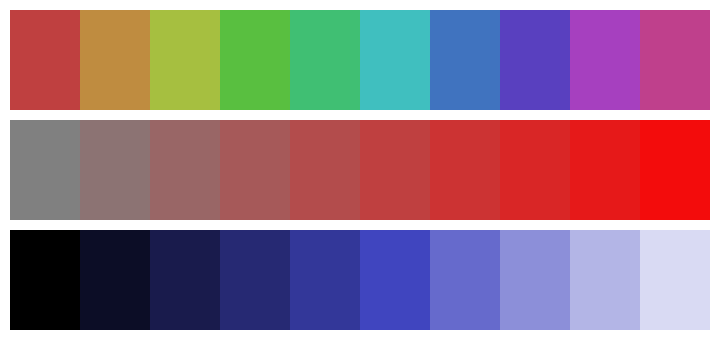To give a little more explanation, the first row has varying hue:

• Hue 0 is red.
• As the hue value increases the colour gradually changes through yellow to green.
• Hue 0.333 is green.
• As the hue value increases the colour gradually changes through cyan to blue.
• Hue 0.666 is blue.
• As the hue value increases the colour gradually changes through magenta back to red.
• Hue is a cyclical quantity. As it moves from 0 to 1 it completes a full red-green-blue colour circle and ends up back at red.

The second row has varying saturation. Each patch has the same red hue, but it changes from grey to more saturated red as the saturation increases from 0 to 0.9.

The third row has varying lightness. Each patch has the same blue hue, but it changes from black to a lighter and lighter blue, eventually moving towards white as lightness increases.

## Transparent colours

You can transparent colours by adding an extra parameter, a, which has a value between 0.0 (totally transparent, ie invisible) and 1.0 (fully opaque). This can be done with any of the above methods:

• RGB colours: Color(r, g, b, a)
• Grey colours: Color(k, a)
• CSS colours: Color(name, a)
• HSL colours: Color.of_hsla(h, s, l, a) (note that this is of_hsla rather than of_hsl).

This code creates a set of 50% transparent coloured squares, using the four functions above. The code first draws a black bar across the image, and paints the transparent colours over the top, so you can see the bar through the squares:

from generativepy.drawing import make_image, setup
from generativepy.color import Color
from generativepy.geometry import Rectangle

def draw_alpha(ctx, width, height, frame_no, frame_count):
setup(ctx, width, height, background=Color(1))

Rectangle(ctx).of_corner_size((10, 50), 450, 20).fill(Color(0))

pos = [20, 10]
w = 100
space =110
h = 100

Rectangle(ctx).of_corner_size(pos, w, h).fill(Color(1.0, 0.5, 0.0, 0.5))
pos += space
Rectangle(ctx).of_corner_size(pos, w, h).fill(Color(0.6, 0.5))
pos += space
Rectangle(ctx).of_corner_size(pos, w, h).fill(Color("tomato", 0.5))
pos += space
Rectangle(ctx).of_corner_size(pos, w, h).fill(Color.of_hsla(0.4, 0.5, 0.5, 0.5))
pos += space

make_image("colour-alpha.png", draw_alpha, 470, 120)


Here is the image it creates: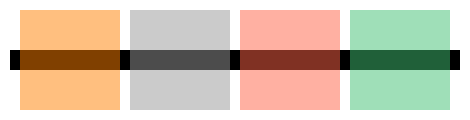## Derived colours

If you have an existing colour col, you can create a new colour col_new based on the existing colour. There are two ways of doing this.

This code creates a new colour with its r value set to 0.5:

col_new = col.with_r(0.5)


This code creates a new colour with its r value multiplied by 1.2:

col_new = col.with_r_factor(1.2)


You can use a factor that is greater than 1 to increase the amount of red in the colour, or a factor that is less than 1 to decrease the amount of red in the colour. But remember:

• If the colour has no red to start with (for instance the RGB colour (0, 1, 0.3), then with_r_factor will have no effect because it is just multiplying 0 by a factor. You can still use with_r to ad red into a colour.
• The total amount of red in the colour is clamped at the maximum, 1.0. Once you hit the limit then increasing the factor won't make the colour any redder.

Similar functions exist for g (green), b (blue), a (alpha), h (hue), s (saturation), and l (lightness). You can apply these functions to any colour. For example, even if a colour was initially defined with RGB values, you can still alter its saturation. Under the hood, the colour will be converted to HSL, modified, then converted back to RGB.

This code illustrates the use of with_g_factor, with_l_factor, and with_s_factor:

from generativepy.drawing import make_image, setup
from generativepy.color import Color
from generativepy.geometry import Rectangle

def draw_delta(ctx, width, height, frame_no, frame_count):
setup(ctx, width, height, background=Color(1))

pos = [10, 10]
w = 100
h = 100

Rectangle(ctx).of_corner_size(pos, w, h).fill(col.with_g_factor(0.8))
pos += w
Rectangle(ctx).of_corner_size(pos, w, h).fill(col)
pos += w
Rectangle(ctx).of_corner_size(pos, w, h).fill(col.with_g_factor(1.2))

pos = [10, 120]

Rectangle(ctx).of_corner_size(pos, w, h).fill(col.with_l_factor(0.6))
pos += w
Rectangle(ctx).of_corner_size(pos, w, h).fill(col)
pos += w
Rectangle(ctx).of_corner_size(pos, w, h).fill(col.with_l_factor(1.4))

pos = [10, 230]

Rectangle(ctx).of_corner_size(pos, w, h).fill(col.with_s_factor(0.6))
pos += w
Rectangle(ctx).of_corner_size(pos, w, h).fill(col)
pos += w
Rectangle(ctx).of_corner_size(pos, w, h).fill(col.with_s_factor(1.4))

make_image("colour-delta.png", draw_delta, 320, 340)


Here is the image it creates: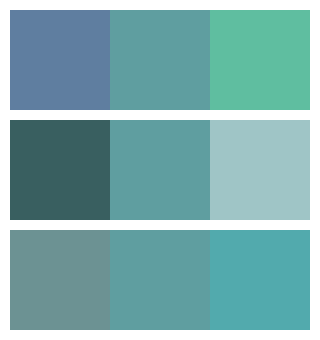The first line of 3 colours shows the colour cadetblue - with green reduced to 80%, unmodified, and with green increased to 120%.

The second line shows the same colour - with lightness reduced to 60%, unmodified, and with lightness increased to 140%.

The third line shows the same colour - with saturation reduced to 60%, unmodified, and with saturation increased to 140%.